Next: Verification of SSC Conditions Up: Sufficient Optimality for Discretized Previous: Known SSC Results for

# Second Order Sufficient Conditions for the Discretized Problems

The control problems (P) and (EB) defined in the previous sections lead after suitable discretization to nonlinear finite-dimensional optimization problems of the form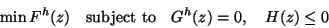(4.1)

where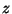comprises the discretized control and state variables.

A discretization (P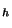) of (P) is given in section 2 while the elliptic problem is assumed to be discretized as described in detail in [16,18].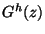symbolizes the state equation and boundary conditions while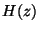denotes both pointwise control and state constraints, the only constraints of inequality type prescribed above. Thus, alternatively, it can be written as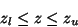(4.2)

where components of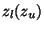are taken as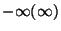if no constraint is imposed on the component.

We state the well-known SSC for (4.1), assuming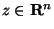,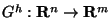,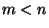. Let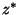be an admissible point satisfying the first-order necessary optimality conditions with associated Lagrange multipliers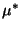and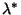. Let further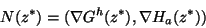be a column-regular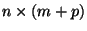matrix where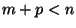and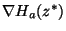denotes the gradients of the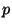active inequality constraints. Let finally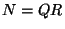be a QR decomposition and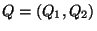a splitting into the first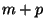and the remaining columns.

The pointis a strict local minimizer if a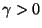exists such that, see, for example,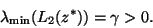(4.3)

Here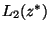is the projected Hessian of the Lagrangian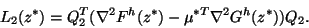No Hessian of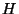appears on the right due to its linearity. Next, we will detail how condition (4.3) will be checked for the discrete versions of both the parabolic and the elliptic control problems. As was already done in [16,18] the control problems are written in the form of AMPL  scripts. This way, a number of nonlinear optimization codes can be utilized for their solution. It had been an observation in our previous work that from the currently available codes only LOQO  is able to solve all the problems effectively and for sufficiently fine discretizations. The following is independent of the solver used.

After computing a solution an AMPL stub (or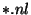) file is written as well as a file with the computed Lagrange multipliers. This allows to check the SSC (4.3) with the help of a Fortran, alternatively, a C or Matlab, program.

This program reads the files and verifies first the necessary first-order optimality conditions, the column regularity of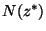and the strict complementarity. For this, it utilizes routines provided by AMPL which permit evaluation of the objective and constraint gradients. Next, the the QR decomposition ofis computed by one of the methods exploiting sparsity. We have utilized the algorithm described in . AMPL also provides a routine to multiply the Hessian of the Lagrangian times a vector. This is called with the columns of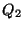and thuscan be formed. Its eigenvalues are computed with LAPACK routine DSYEV and the smallest eigenvalue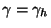is determined. The use of this eigenvalue routine is possible since the order of the matrices corresponding to the "free" control variables is moderate. In case of distributed control problems when this number may be on the order of the state variables, a sparse solver, preferably just for finding the minimal eigenvalue, will have to be used.Next: Verification of SSC Conditions Up: Sufficient Optimality for Discretized Previous: Known SSC Results for
Hans Mittelmann
2000-08-31